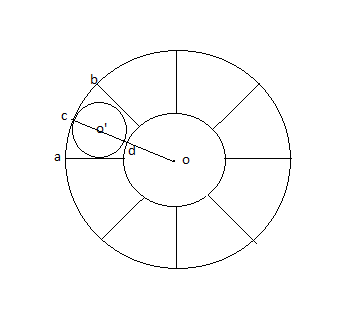# Circle, Circle, Circle...math10Intra AUST Programming Co...
Limits 1s, 512 MBThere are 2 circles with the radius od = r and oc = R and they are sharing a common center o.

The space between the two circles is cut into n sections (the given image has 8 sections). You need to determine the maximum radius of the circle that can be drawn in each section with these rules,

1. The circle needs to be inside the section, will not intersect with another section or the smaller circle.

2. o’ is the center of the circle you are going to draw

3. c is the center of the acb arc

4. The line oo’c should be a straight line

## Input

Input starts with an integer T (T <= 100) denoting the number of test cases.

Each case starts with a line containing 3 integer R, r, n

1 <= r < R <= 1000

1 <= n <= 180

## Output

For each case print the maximum radius of the circle that can be drawn in each section on each line.

Errors less than $10^-6$ will be ignored.

## Sample

InputOutput
1
10 5 5


2.5


### Submit